## 2.1  Rotational Kinetic Energy

We consider a rigid body to be made up of a large number of constituent particles with mass m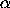, position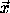, and velocities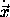, with rigid positional constraints among them. The kinetic energy is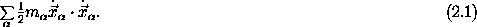It turns out that the kinetic energy of a rigid body can be separated into two pieces: a kinetic energy of translation and a kinetic energy of rotation. Let's see how this comes about.

The configuration of a rigid body is fully specified given the location of any point in the body and the orientation of the body. This suggests that it would be useful to decompose the position vectors for the constituent particles as the sum of the vector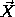to some reference position in the body and the vector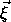from the reference position to the particular constituent element with index: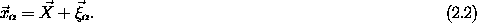Along paths, the velocities are related by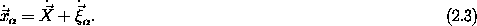So in terms of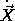and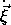the kinetic energy is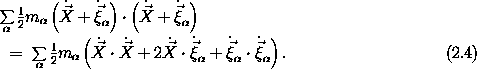If we select the reference position in the body to be its center of mass,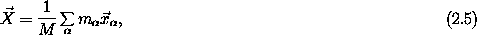where M = summis the total mass of the body, then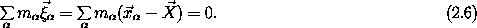So along paths the relative velocities satisfy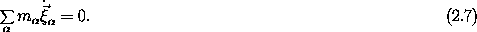The kinetic energy is then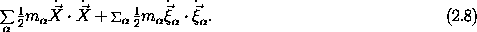The kinetic energy is the sum of the kinetic energy of the motion of the total mass at the center of mass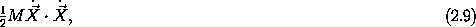and the kinetic energy of rotation about the center of mass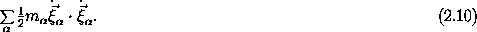Written in terms of appropriate generalized coordinates, the kinetic energy is a Lagrangian for a free rigid body. If we choose generalized coordinates so that the center of mass position is entirely specified by some of them and the orientation is entirely specified by others, then the Lagrange equations for a free rigid body will decouple into two groups of equations, one concerned with the motion of the center of mass and one concerned with the orientation.

Such a separation might occur in other problems, such as a rigid body moving in a uniform gravitational field, but in general, potential energies cannot be separated as the kinetic energy separates. So the motion of the center of mass and the rotational motion are usually coupled through the potential. Even in these cases, it is usually an advantage to choose generalized coordinates that separately specify the position of the center of mass and the orientation.